# Merge two sorted linked lists such that the merged list is in reverse order

Medium

Microsoft

### Three Solutions Discussed

The problem would have been quite easy if arrays would be in place of the linked lists because accessing an element in an array is much easier. Well, we have gone through the following solutions in this blog.

• Broute force approach 1 — Reversing both the list and merging
• Brute force approach 2 — Merging both the list and reversing
• Iterative approach — Single Scan using the merge function

#### Key Takeaway after reading this blog

This is a good problem to understand the application of reversing and merging to solve a linked list problem. We use these ideas to solve several other interview problems in the linked list.

#### Let’s understand the problem

Given two sorted linked lists of size m and n respectively, write a program to merge both such that the resulting list becomes in decreasing order.

Examples

Input: list1: 8->10->20->35, list2: 12->30->50

Output: res: 50->35->30->20->12->10->8

Input: list1: 7->15->20, list2: 20->25

Output: res: 25->20->20->15->7

## Brute force approach 1: Reversing both the list and merging

If we reverse both linked lists and merge them in the same format (decreasing), we get the answer.

#### Algorithm Pseudo Code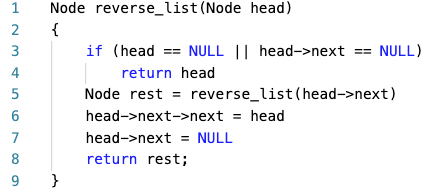Merge two linked list in decreasing order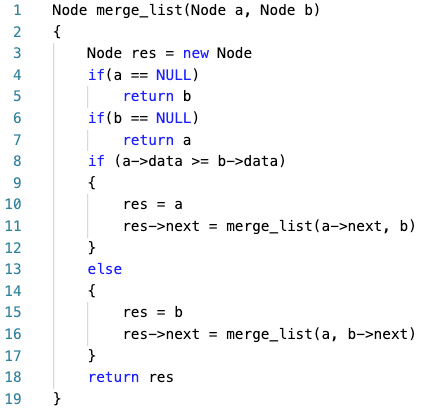Complexity Analysis

The overall time complexity of reversing =O(n) +O(m) = O(n+m) (Think!)

The time complexity of merging both the lists = O(n+m) (Think!)

Overall time complexity = The time complexity of reversing + The time complexity of merging = O(n+m) + O(n+m) = O(n+m)

Space Complexity = O(n + m), due to recursion stacks of reversing both the linked list.

## Brute force approach 2: Merging both the list and reversing

This approach is very similar to the previous one. Just we have to change the order of the steps. So, first, do the merging followed by the reversing of the resulting list.

#### Algorithm Pseudo Code

merge two linked list in increasing order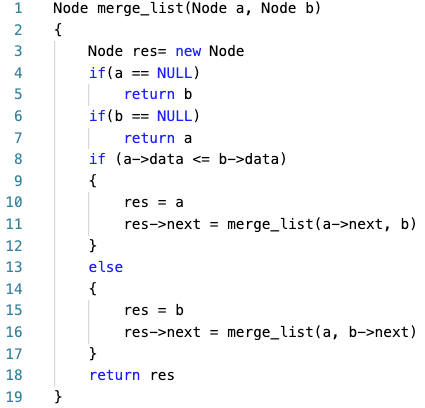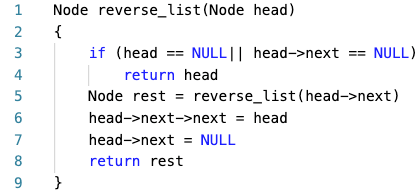Complexity Analysis

Overall time complexity = The time complexity of reversing + The time complexity of merging = O(n+m) + O(n+m) = O(n+m)

Space Complexity = O(n + m), due to recursion stacks of reversing both the linked list.

## 3. Iterative approach - Single scan using the merge function

This is an efficient solution and it does the works in O(1) space and linear time. Idea is to traverse both the lists simultaneously and insert the minimum of both elements (of both lists) at the beginning of the new list. If only one list remains non-empty insert all elements of the list into the new list.

#### Algorithm Pseudo Code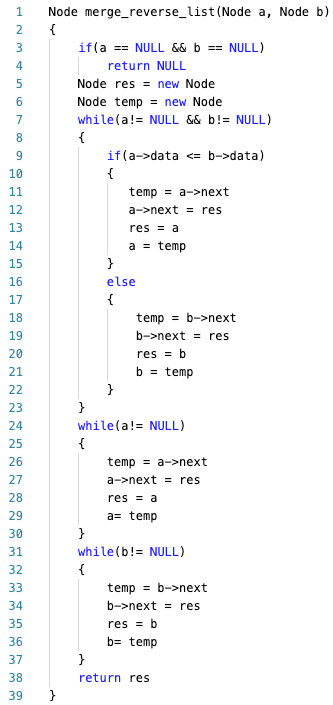Complexity Analysis

We are traversing each node once in both the list.

Time Complexity = O(m + n), due to loops iterations.

Space Complexity =O(1), as no extra space has been used.

### Similar Questions To Solve

Enjoy learning, enjoy algorithm!

☆ 16-Week Live DSA Course
☆ 10-Week Live DSA Course